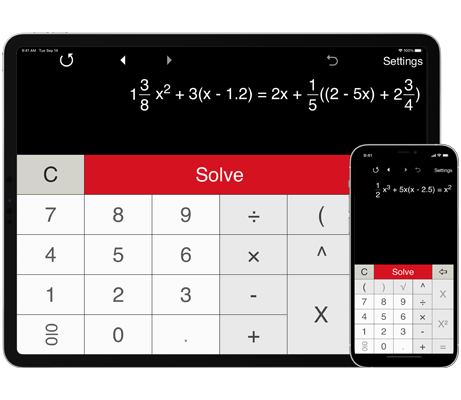# Linear equation calculator

Solves linear equations in one variable, including linear equations with parentheses, fractions, and decimals.
Shows step-by-step solution.

## Linear equation calculator with steps

Linear equation calculator helps you solve a wide range of linear equations (first degree equations) in one variable, including linear equations with parentheses, fractions, and decimals.

Solving one-step linear equations with decimals and fractions.

Solving two-step linear equations with decimals and fractions.

Solving multi-step linear equations with decimals and fractions.

Pre-defined formats for solving first-degree equations in the form ax + b = c and ax + b = cx + d.